# GSEB Solutions Class 12 Statistics Part 2 Chapter 1 Probability Ex 1

Gujarat Board Statistics Class 12 GSEB Solutions Part 2 Chapter 1 Probability Ex 1 Textbook Exercise Questions and Answers.

## Gujarat Board Textbook Solutions Class 12 Statistics Part 2 Chapter 1 Probability Ex 1

Section A

Answer the following questions by selecting a correct option from the given options:

Question 1.
Which event is given by a special subset Φ of the sample space U ?
(a) Certain event
(b) Complementary event of Φ
(c) Union of events U and Φ
(d) Impossible event
(d) Impossible eventQuestion 2.
What is the value of P(A ∩ A’) for events A and A’ ?
(a) 1
(b) 0
(c) 0.5
(d) between 0 and 1
(b) 0

Question 3.
Which of the following options is true for any event of the sample space ?
(a) P(A) < 0 (b) 0 ≤ P(A) ≥ 1 (c) 0 ≤ P(A) ≤ 1 (d) P(A) > 1
(c) 0 ≤ P(A) ≤ 1

Question 4.
Which of the following options is not true for any two events A and B in the sample space U; where, A ⊂ B?
(a) P(A ∩ B) = P(B)
(b) P(A ∩ B) = P(A)
(c) P(A ∪ B) ≥ P(A)
(d) P(B-A) = P(B)-P(A)
(a) P(A ∩ B) = P(B)

Question 5.
What is the other name of the classical definition of probability?
(a) Mathematical definition
(b) Axiomatic definition
(c) Statistical definition
(d) Geometric definition
(a) Mathematical definition

Question 6.
Which of the following statement for probability of elementary events H and T of random experiment of tossing a balanced coin is not true ?
(a) P(T) = 0.5
(b) P(H) + P(T) = 1
(c) P(H ∩ T) = 0.5
(d) P(H) = 0.5
(c) P(H ∩ T) = 0.5Question 7.
Which random experiment from the following random experiments has an infinite sample space ?
(a) Throwing two dice
(b) Selecting two employees from an office
(c) To measure the life of electric bulb
(d) Select a card from 52 cards
(c) To measure the life of electric bulb

Question 8.
If A ∪ A’ = U, then what type of events are A and A’ ?
(a) Independent events
(b) Complementary events
(c) Certain events
(d) Impossible events
(b) Complementary events

Question 9.
If P(A|B) = P(A) and P(B|A) = P(B), then what type of events are A and B ?
(a) Independent events
(b) Complementary events
(c) Certain events
(d) Impossible events
(a) Independent events

Question 10.
Two events A and B of a sample space are mutually exclusive. Which of the following will be equal to P(B-A)?
(a) P(A)
(b) P(B)
(c) P(A ∩ B)
(d) P(A ∪ B)
(b) P(B)Question 11.
What is the total number of sample points in the sample space formed by throwing three six-faced balanced dice simultaneously?
(a) 62
(b) 36
(c) 6 × 3
(d) 63
(d) 63

Question 12.
If one number is randomly selected between 1 and 20, what is the probability that the number is a multiple of 5 ?
(a) $$\frac{1}{2}$$
(b) $$\frac{1}{6}$$
(c) $$\frac{1}{5}$$
(d) $$\frac{1}{3}$$
(b) $$\frac{1}{6}$$

Question 13.
If events A and B are independent, which of the following options is true?
(a) P(A ∩ B) = P(A) × P(B)
(b) P(A ∪ B) = P(A) + P(B)
(c) P(A ∪ B) = P(A) × P(B)
(d) P(A ∩ B) = P(A) + P(B)
(a) P(A ∩ B) = P(A) × P(B)

Question 14.
What is the probability of having 5 Thursdays in the month of February in a year which is not a leap year?
(a) 0
(b) $$\frac{1}{7}$$
(c) $$\frac{2}{7}$$
(d) $$\frac{3}{7}$$
(a) 0Question 15.
If P(A) = 0.4 and P(B’) = 0.3 for two independent events A and B of a sample space, then state the value of P(A ∩ B).
(a) 0.12
(b) 0.42
(c) 0.28
(d) 0.18
(c) 0.28

Question 16.
For two events A and B of a sample space, state the event (A ∩ B) ∪ (A ∩ B’).
(a) Φ
(b) B
(c) A
(d) ∪
(c) A

Question 17.
According to the mathematical definition of probability, what is the probability of each outcome among the n outcomes of a random experiment ?
(a) 0
(b) $$\frac{1}{n}$$
(c) 1
(d) cannot say
(b) $$\frac{1}{n}$$

Section B

Answer the following questions in one sentence:

Question 1.
Give two examples of random experiment.
Two examples of random experiment are:

1. The experiment of throwing a balanced die and
2. The experiment of finding defective units from a lot of units produced.Question 2.
Draw the Venn diagram for A – B, the difference event of A and B.
The Venn diagram for difference event A-B: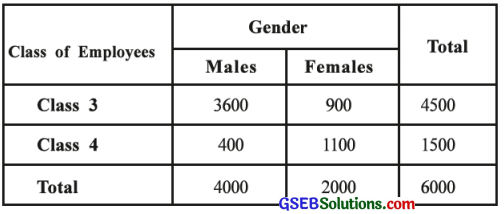Question 3.
Define an event.
Any subset of the sample space of a random experiment is called an event. It is denoted by A, B, C ….

Question 4.
Write the sample space of a random experiment of throwing one balanced die and a balanced coin simultaneously.
The sample space of a random experiment of throwing one balanced die and a balanced coin simultaneously is obtained as follows:
U = {(1, H), (2, H), (3, H), (4, H), (5, H), (6, H), (1, T), (2, T), (3, T), (4, T), (5, T), (6, T)}
Where, H = Head; T = Tail;
1, 2, 3, 4, 5, 6 = Numbers on die.Question 5.
Define conditional probability.
U is a finite sample space and A and B are any two events of U. The probability of event B, under the condition that event A is happened, is called conditional probability of the event B.

Question 6.
State the formula for the probability of occurrence of at least one event out of three events A, B and C.
The formula for the probability of occurrence of at least one event out of three events A, B and C is as follows:
P(A ∪ B ∪ C) = P(A) + P(B) + P(C) – P(A ∩ B) – P(A ∩ C) – P(B ∩ C) + P(A ∩ B ∩ C)

Question 7.
Define independent events.
U is a finite sample space and A and B are any two events of U. If the probability of occurrence of the event A does not depend on the occurrence or non-occurrence of the event B, then the events A and B are called independent events.

Question 8.
Write the law of multiplication of probability for two independent events A and B in a sample space.
The law of multiplication of probability for two independent events A and B in a sample space is as follows:
P(A ∩ B) = P(A) × P(B)

Question 9.
Interpret P(A|B) and P(B|A).
Interpretation of P(A|B): The conditional probability of the event A under the condition that the event B is occurred.
Interpretation of P(B|A): The conditional probability of the event B under the condition that the event A has occurred.Question 10.
When can we say that three events A, B and C in a sample space are exhaustive?
When P(A) + P(B) + P(C) = 1, we can say that three events A, B and C in a sample space are exhaustive.

Question 11.
Arrange P(A ∪ B), P(A), P(A ∩ B), 0, P (A) + P (B) in the ascending order.
0, P(A ∩ B), P(A), P(A ∪ B), P(A) + P(B) are in the ascending order.

Question 12.
Define:
1. Random Experiment: The experiment which can be independently repeated under identical conditions and all its possible outcomes are known but it cannot be predicted with certainty which of the outcomes will appear is called a random experiment.

2. Sample Space: The set of all possible outcomes of a random experiment is called a sample space of that random experiment. It is denoted by U or S.

3. Equi-probable Events: If there is no apparent reason to believe that out of one or more events of a random experiment, any one event is more or less likely to occur than the other events, then those events are called as equi-probable events.

4. Favourable Outcomes: If some outcomes out of all the elementary outcomes in the sample space of a random experiment indicate the occurrence of a certain event A, then these outcomes are called the favourable outcomes of the event A.

5. Probability (Mathematical Definition): If out of n outcomes of the finite sample space of a random experiment which are mutually exclusive, exhaustive and equi-probable, m outcomes are favourable for an event A, then the probability of the event A, P(A) = $$\frac{m}{n}$$.

6. Probability (Statistical Definition) :
Suppose, a random experiment is repeated n times under identical conditions. If an ‘event A occurs in m trials then the relative frequency $$\frac{m}{n}$$ of the event A gives the estimate of the probability of the event A. When n tends to infinity, the limiting value of $$\frac{m}{n}$$ is called the probability of the event A. Thus,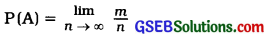7. Impossible Event: The special subset Φ or { } of the sample space of a random experiment is called an impossible event.

8. Certain Event: The special subset U of the sample space of a random experiment is called a certain event.Question 13.
For two events A and B in a sample space, A ∩ B = Φ and A ∪ B = U. State the values of P(A ∩ B) and P(A ∪ B).
A ∩ B = Φ and A ∪ B = U
∴ P(A ∩ B) = 0 and P(A ∪ B) = 1

Question 14.
If two events A and B in a sample space are independent, then state the formula for P(A ∪ B).
A and B are independent events.
∴ P(A ∪ B) = P(A) + P(B) – P(A ∩ B)
= P(A) + P(B) – [P(A) ∙ P(B)]

Question 15.
If A = {x|0 < x < 1} and B = {x|$$\frac{1}{4}$$ ≤ x ≤ 3}, then find A n B.
A = {x|0 < x < 1} = {$$\frac{1}{4}, \frac{1}{2}, \frac{3}{4}$$}
B = {x|$$\frac{1}{4}$$ ≤ x ≤ 3} = {$$\frac{1}{4}, \frac{1}{2}, \frac{3}{4}$$, ……, 1, 2, 3}
∴ A ∩ B = {x|$$\frac{1}{4}$$ ≤ x < 1}

Question 16.
For two independent events A and B, P (A) = 0.5 and P(B) = 0.7. Find P(A’ ∩ B’).
A and B are independent events. So A’ and B’ are also independent events. P(A) = 0.5, P(B) = 0.7 are given.
∴ P(A’ ∩ B’) = P(A’) ∙ P(B’)
= [1 – P(A)] [1 – P(B)]
= [1 – 0.5] [1 – 0.7]
= [0.5 × 0.3]
= 0.15

Question 17.
If P(A) = 0.8 and P (A ∩ B] = 0.25, find P(A – B).
P(A) = 0.8, P(A ∩ B) = 0.25 are given.
∴ P (A – B) = P(A) – P(A ∩ B)
= 0.8 – 0.25 = 0.55Question 18.
If P(A) = 0.3 and P(A ∩ B) = 0.03, find P(B|A).
P(A) = 0.3, P(A ∩ B) = 0.03 are given.
∴ P(B|A) = $$\frac{P(A \cap B)}{P(A)}$$ = $$\frac{0.03}{0.3}$$ = 0.1

Question 19.
If P(A) = P(B) = K for two mutually exclusive events A and B, find P(A ∪ B).
P (A) = P(B) = k.
A, B are mutually exclusive events
∴ P(A ∩ B) = 0
Now, P(A ∪ B) = P(A) + P(B) .
= k + k = 2k

Question 20.
If P(A’ ∩ B) = 0.45 and A ∩ B = Φ, find P(B).
P (A’ ∩ B) = 0.45 and A ∩ B = Φ are given.
∴ P(A ∩ B) = 0
Now, P(A’ ∩ B) = P(B) – P(A ∩ B)
∴ 0.45 = P(B) – 0
∴ P(B) = 0.45

Question 21.
Two events A and B in a sample space are mutually exclusive and exhaustive. If P(A) = $$\frac{1}{3}$$, find P(B).
A and B are mutually exclusive and exhaustive events.
∴ P(A) + P(B) = 1 (Putting P(A) = $$\frac{1}{3}$$)
$$\frac{1}{3}$$ + P(B) = 1
∴ P(B) = 1 – $$\frac{1}{3}$$ = $$\frac{2}{3}$$Question 22.
2 % items in a lot are defective. What is the probability that an item randomly selected from this lot is non-defective?
A = Event that an item is defective
∴ P(A) = 2 % = $$\frac{2}{100}$$ = 0.02
A’ = Event that an item is non-defective
∴ P(A’) = 1 – P(A) = 1 – 0.02 = 0.98

Question 23.
State the number of sample points in the random experiment of tossing five balanced coins.
The number of sample points in the random experiment of tossing five balanced coins is n = 25 = 32.

Question 24.
State the number of sample points in the random experiment of tossing one balanced coin and two balanced dice simultaneously.
The number of sample points in the random experiment of tossing one balanced coin and two balanced dice simultaneously is n = 21 × 62 = 2 × 36 = 72.

Question 25.
Is it possible that P (A) = 0.7 and P (A ∪ B) = 0.45 for two events A and B in a sample space ?
P (A) = 0.7 and P(A ∪ B) = 0.45 is not possible because P(A ∪ B) < P(A).

Question 26.
Two cards are selected one by one with replacement from 52 cards. State the number of elements in the sample space of the random experiment.
The number of elements in the sample space of this random experiment of drawing two cards one by one with replacement from 52 cards is n = 52C1 × 52C1 = 52 × 52 = 2704

Question 27.
For two independent events A and B, P(B|A) = $$\frac{1}{2}$$ and P(A ∩ B) = $$\frac{1}{5}$$. Find P(A).
P(B|A) = $$\frac{1}{2}$$, P(A ∩ B) = $$\frac{1}{5}$$, P(A) = ?
P(B|A) = $$\frac{P(A \cap B)}{P(A)}$$
∴ $$\frac{1}{2}$$ = $$\frac{\frac{1}{5}}{\mathrm{P}(\mathrm{A})}$$
∴ $$\frac{1}{2}$$p(A) = $$\frac{1}{5}$$
∴ P(A) = $$\frac{1}{5}$$ × 2 = $$\frac{2}{5}$$

Question 28.
1998 tickets out of 2000 tickets do not have a prize. If a person randomly selects one ticket from 2000 tickets, then what is the probability that the ticket selected is eligible for prize ?
Total number of tickets = 2000
No. of tickets do not have a prize = 1998
No. of tickets eligible for prize = 2000 – 1998
= 2
Total number of outcomes of selecting a ticket is n = 2000c1 = 2000
A = Event that the selected ticket is eligible for prize
∴ m = 2c1 = 2
∴ P(A) = $$\frac{m}{n}$$
= $$\frac{2}{2000}$$
= $$\frac{1}{1000}$$Section C

Answer the following questions as required:

Question 1.
Define the following events and draw their Venn diagram:
1. Mutually exclusive events
2. Union of events
3. Intersection of events
4. Difference event
5. Exhaustive events
6. Complementary event
1. Mutually exclusive events: Suppose, U is a finite sample space. A and B are any two events of U. If A ∩ B = Φ, then A and B are mutually exclusive events.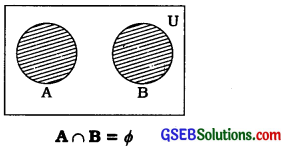2. Union of events: Suppose, U is a finite sample space. A and B are any two events of U. Event A occurs or event B occurs or events A and B occur togethers, i.e., at least one of the events A and B occurs is called the union of events A and B. It is denoted by A ∪ B. Thus,
A ∪ B = {x; x ∈ A or x ∈ B or x ∈ A ∩ B}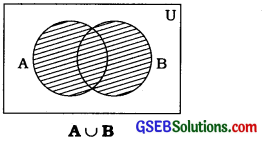3. Intersection of Events: Suppose, U is a finite sample space. A and B are any two events of U. The event that A and B occur together is called the intersection of events A and B. It is denoted by A ∩ B. Thus,
A ∩ B = {x; x ∈ A and x ∈ B}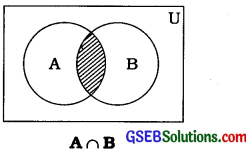4. Difference event: Suppose, U is a finite sample space. A and B are any two events of U. The event that A occurs but B does not occur is called the difference event of A and B. It is denoted by A – B or A ∩ B’. Similary, the event that A does not occur but B occurs is called the difference event of B and A. It is denoted by B – A or A’ ∩ B. Thus,
A – B = {x; x ∈ A and x ∉ B}
B – A = {x; x ∈ B and x ∉ A}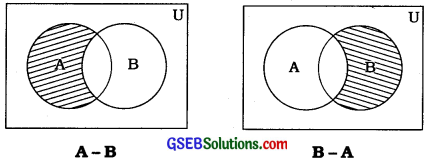5. Exhaustive events: Suppose, U is a finite sample space. A and B are any two events of U. If A ∪ B = U, then A and B are called exhaustive events.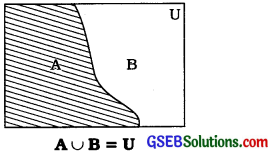6. Complementary event: Suppose, A is any event of the finite sample space U. The event that A does not occur means the event consists of elements in U but not in A is called the complementary event of A. It is denoted by A’. Thus,
A’ = {x; x ∉ A, x ∈ U}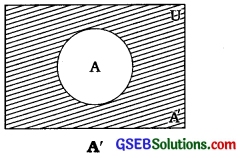Question 2.
Give the illustrations of finite and infinite sample space.
Finite sample space: The sample space obtained for the random experiment throwing a balanced’ six face die U = {1, 2, 3, 4, 5, 6} is the illustration of finite sample space.

Infinite sample space: The sample space obtained for the random experiment of selecting a card from a pack of 52 cards till it is ace of heart is the illustration of infinite sample space.

Question 3.
Give the illustrations of impossible and certain event.
Impossible event: Event to get the number greater than 6 on the upper side of a balanced die.
Certain event: Event to get head or tail in tossing a balanced coin.

Question 4.
State the characteristics of random experiment.
The characteristics of random experiment are as follows :

• It can be repeated under identical conditions,
• Its all possible outcomes are known,
• It cannot be predicted with certainty which outcome will appear and
• It results into a certain outcome.

Question 5.
State the assumptions of mathematical definition of probability.
The assumptions of mathematical definition of probability are as follows:

• The number of outcomes in the sample space is finite.
• The number of all possible outcomes of the sample space is known.
• The outcomes of the sample space are equi-probable.

Question 6.
State the limitations of mathematical definition of probability.
The limitations of mathematical definition of probability are as follows:

• The probability of an event cannot be found if the outcomes are infinite.
• If the total number of outcomes is not known, the probability of an event cannot be found.
• If the elementary outcomes in the sample space are not equi-probable, the probability of an event cannot be found.Question 7.
State the limitations of statistical definition of probability.
The limitations of statistical definition of probability are as follows:

• The infinite value of n cannot be taken in practice.
• The exact value of probability cannot be known.

Question 8.
Explain the equi-probable events with illustration.
If there is no apparant reason to believe that out of one or more events of a random experiment, any one event is more or less likely to occur than the other events, then those events are called equi-probable.

Illustration: In the random experiment of tossing a balance coin, two events of getting head (H) and getting tail (T) are equi-probable, because P (H) = P (T) = $$\frac{1}{2}$$

Question 9.
State the law of addition of probability for two events A and B. Write the law of addition of probability if these two events are mutually exclusive.
The law of addition of probability for two events A and B is as follows :
P(A ∪ B) = P(A) + P(B) – P(A ∩ B)
If A and B are mutually exclusive events, A ∩ B = 0 and P (A ∩ B) = 0. Hence, the law of addition of probability for two events A and B is written as follows:
P(A ∪ B) = P(A) + P(B)

Question 10.
State the law of multiplication of probability for two events A and B. Write the law of multiplication of probability if these two events are independent.
The law of multiplication of probability for two events A and B is as follows:
P(A ∩ B) = P(A|B) ∙ P(B) OR
P(A ∩ B) = P(B|A) ∙ P(A)
If two events A and B are independent, P(A|B) = P(A) and P(B|A) = P(B). So the law of multiplication of probability for two events A and B is written as follows:
P(AnB) = P(A) ∙ P(B)Question 11.
State the following results for two independent events A and B:
1. P(A ∩ B)
2. P (A’ ∩ B’)
3. P(A ∩ B’)
4. P(A’ ∩ B)
For two independent events A and B, the results are obtained as follows:
1. P(A ∩ B) = P(A) × P(B)
2. P(A’ ∩ B’) = P(A’) × P(B’)
3. P(A ∩ B’) = P(A) × P(B’)
4. P(A’ ∩ B) = P(A’) × P(B) .

Question 12.
If p(A) = $$\frac{1}{3}$$, P(B) = $$\frac{2}{3}$$ and P(A ∩ B) = $$\frac{1}{6}$$, then find P(A’ ∩ B’).
p(A) = $$\frac{1}{3}$$, P(B) = $$\frac{2}{3}$$ and P(A ∩ B) = $$\frac{1}{6}$$ are given.
According to the law of addition of probability,
P(A ∪ B) = P(A) + P(B) – P(A ∩ B)
= $$\frac{1}{3}+\frac{2}{3}-\frac{1}{6}$$
= $$\frac{2+4-1}{6}$$
= $$\frac{5}{6}$$
Now, P(A’ ∩ B’) = P(A ∪ B)’
= 1 – P(A ∪ B)
= 1 – $$\frac{5}{6}$$
= $$\frac{6-5}{6}$$
= $$\frac{1}{6}$$

Question 13.
If P(B) = 2P(A|B) = 0.4, then find P(AnB).
Ans.
P(B) = 2P(A|B) = 0.4
∴ P(B) = 0.4, 2P(A|B) = 0.4
∴ P(A|B) = $$\frac{0.4}{2}$$ = 0.2
Now, P(A|B) = $$\frac{P(A \cap B)}{P(B)}$$
∴ P(A ∩ B) = P(A|B) ∙ P(B)
= 0.2 × 0.4 = 0.08Question 14.
If the events A and B are independent and 3P(A) = 2P(B) = 0.12, then find P(A ∩ B).
3P(A) = 2P(B) = 0.12
∴ 3P(A) = 0.12 and 2P(B) = 0.12
∴ P(A) = $$\frac{0.12}{3}$$
= 0.04
and
P(B) = $$\frac{0.12}{2}$$
= 0.06
Now, A and B are independent events.
∴ P(A ∩ B) = P(A) – P(B)
= 0.04 × 0.06
= 0.0024

Question 15
If 5P (A) = 3P (B) = 2P (A ∪ B) = f for two events A and B, then find P(A’ ∪ B’).
5P (A) = 3P(B) = 2P (A ∪ B) = $$\frac{3}{2}$$
∴ 5P(A) = $$\frac{3}{2}$$ and 3P(B) = $$\frac{3}{2}$$ and 2P(A ∪ B) = $$\frac{3}{2}$$
∴ P(A) = $$\frac{3}{2 \times 5}$$ = $$\frac{3}{10}$$
∴ P(B) = $$\frac{3}{2 \times 3}$$ = $$\frac{1}{2}$$
∴ P(A ∪ B) = $$\frac{3}{2 \times 2}$$ = $$\frac{3}{4}$$
According to the law of addition of probability,
P(A ∪ B) = P(A) + P(B) – P(A ∩ B)
$$\frac{3}{4}$$ = $$\frac{3}{10}+\frac{1}{2}$$ – P(A ∩ B)
∴ P(A ∩ B) = $$\frac{3}{10}+\frac{1}{2}-\frac{3}{4}$$
= $$\frac{6+10-15}{20}$$ = $$\frac{1}{20}$$
Now, P(A’ ∪ B’) = P(A ∩ B)’
= 1 – P(A ∩ B) = 1 – $$\frac{1}{20}$$ = $$\frac{19}{20}$$Question 16.
If P(A ∩ B) = 0.12 and P(B) = 0.3 for two independent events A and B, then find P(AuB).
A and B are independent events.
∴ P(A ∩ B) = P(A) – P(B)
∴ 0.12 = P(A) × 0.3
∴ P(A) = $$\frac{0.12}{0.3}$$ = 0.4
Now, according to the law of addition of probability,
P(A ∪ B) = P(A) + P(B) – P(A ∩ B)
= 0.4 + 0.3 – 0.12
= 0.58

Question 17.
If A = {x| 1 < x < 3} and B = {x|$$\frac{1}{2}$$ ≤ x < 2}, then find A ∪ B and A ∩ B.
A = {x| 1 < x < 3} and B = {x|-|- =£ x < 2}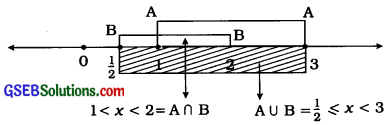From the above diagram, the events are obtained as follows:
(1) A ∪ B = {x|$$\frac{1}{2}$$ ≤ x < 3}
(2) A ∩ B = {x|1 < x < 2}

Question 18.
The probability of occurrence of at least one of the two events A and B is The probability that event A occurs, but event B does not occur is Find the probability of event B.
A ∪ B = Event that at least one of the two events A and B occurs
∴ P(A ∪ B) = $$\frac{1}{4}$$
AnB’ = Event that A occurs, but B does not occur
∴ P(A ∩ B’) = $$\frac{1}{5}$$
According to the law of addition of probability,
P(A ∪ B) = P(A) + P(B) – P(A ∩ B)
∴ P(A ∪ B) = P(A) – P(A ∩ B) + P(B)
[Putting, P(A ∩ B’) = P(A) – P(A n B)]
∴ P(A ∪ B) = P(A ∩ B’) + P(B)
∴ P(B) = P(A ∪ B) – P(A ∩ B’)
= $$\frac{1}{4}-\frac{1}{5}$$
= $$\frac{5-4}{20}$$
= $$\frac{1}{20}$$Question 19.
If P(B) = $$\frac{3}{5}$$ and P(A’ ∩ B) = $$\frac{1}{2}$$ for two events A and B, find P(A|B).
P(B) = $$\frac{3}{5}$$ and P(A’ ∩ B) = $$\frac{1}{2}$$ are given.
∴ P(A’ ∩ B) = P(B) – P(A ∩ B)
∴ $$\frac{1}{2}$$ = $$\frac{3}{5}$$ – P(A ∩ B)
∴ P(A ∩ B) = $$\frac{3}{5}$$ – $$\frac{1}{2}$$ = $$\frac{6-5}{10}$$ = $$\frac{1}{10}$$
Now, P(A|B) = $$\frac{P(A \cap B)}{P(B)}$$
= $$\frac{1}{\frac{10}{\frac{3}{5}}}$$ = $$\frac{1}{10} \times \frac{5}{3}$$ = $$\frac{1}{6}$$
∴ P(A|B) = $$\frac{1}{6}$$

Question 20.
6 persons have a passport in’ a group of 10 persons. If 3 persons are randomly selected from this group, find the probability that
(i) all the three persons have a passport
(ii) two persons among them do not have a passport.
Out of 10 persons, 6 persons have a passport. So 4 persons do not have a passport.
Now, 3 persons out of 10 persons are randomly selected.
∴ Total number of primary outcomes,
n = 10C3 = $$\frac{10 \times 9 \times 8}{3 \times 2 \times 1}$$ = 120

(i) A = Event that all three persons have a passport
∴ Favourable outcomes for the event A is
m = 6C3 = $$\frac{6 \times 5 \times 4}{3 \times 2 \times 1}$$ = 120
P (A) = $$\frac{m}{n}$$ = $$\frac{20}{120}$$ = $$\frac{1}{6}$$

(ii) B = Event that two persons among three persons do not have a passport
∴ Favourable outcomes for the event B is
m = 6C1 × 4C2 = 6 × 6 = 36
∴ P(B) = $$\frac{m}{n}$$ = $$\frac{36}{120}$$ = $$\frac{3}{10}$$

Question 21.
The probability that the tax-limit for income of males increases in the budget of a year is 0.66 and the probability that the tax- limit increases for income of females is 0.72. The probability that the tax-limit increases for income of both the males and females is 0.47. Find the probability that
(i) the tax-limit increases for income of only one of the two, males and females,
(ii) the tax-limit does not increase for income of males as well as females in the budget of that year.
A = Event that the tax-limit for income of males increases.
B = Event that the tax-limit for income of females increases.
A ∩ B = Event that the tax-limit for income of male and female increases.
Here, P(A) = 0.66, P(B) = 0.72 and P(A ∩ B) =0.47 are given.

(i) C = Event that the tax-limit increases only for one of the two male and female.
Event C occurs in following two ways:
→ A ∩ B’ = Event that the tax-limit increases for the income of male only and not of female OR
→ A’ ∩ B = Event that the tax-limit increases for the income of female only and not of male Events A ∩ B’ and A’ ∩ B are mutually exclusive,
∴ P(C) = P(A ∩ B’) + P(A’ ∩ B)
= [P(A) – P(A ∩ B)l + (P(B) – P(A ∩ B)]
= [0.66 – 0.47] + [0.72 – 0.47]
= 0.19 + 0.25 = 0.44

(ii) A’ ∩ B’ = Event that the tax-limit does not increase for income of either male or female.
Now, P(A ∪ B) = P(A) + P(B) – P(A ∩ B)
= 0.66 + 0.72 – 0.47 = 0.91
∴ P(A’ ∩ B’) = P(A ∪ B)’ = 1 – P(A ∪ B)
= 1 – 0.91 = 0.09Question 22.
The price of petrol rises in 80 % of the cases and the price of diesel rises in 77% of the cases after the rise in price of crude oil. The price of petrol and diesel rises in 68 % cases. Find the probability that the price of diesel rises under the condition that there is a rise in the price of petrol.
A = Event that the price of petrol rises
∴ P(A) = $$\frac{80}{100}$$
B = Event that the price of diesel rises
∴ P(B) = $$\frac{77}{100}$$
A ∩ B = Event that the prices of both petrol and diesel rises
∴ P(A ∩ B) = $$\frac{68}{100}$$
B|A = Event that the price of diesel rises knowing that the price of petrol rises
∴ P(B|A) = $$\frac{P(A \cap B)}{P(A)}$$
= $$\frac{\frac{68}{100}}{\frac{80}{100}}$$
= $$\frac{68}{100} \times \frac{100}{80}$$
= $$\frac{17}{20}$$

Question 23.
As per the prediction of weather bureau, the probabilities for rains on three days; Thursday, Friday and Saturday in the next week are 0.8, 0.7 and 0.6 respectively. Find the probability that it rains on at least one of the three days in the next week.
(Note:The events of rains on three days; Thursday, Friday and Saturday of a week are independent.)
A = Event that it rains on Thursday
B = Event that it rains on Friday
C = Event that it rains on Saturday
Here, P(A) = 0.8, P(B) = 0.7 and P(C) = 0.6 are given.
A, B and C are independent events.
∴ P(A ∩B) = P(A) ∙ P(B) = 0.8 × 0.7 = 0.56
P(A ∩ C) = P(A) ∙ P(C) = 0.8 × 0.6 = 0.48
P(B ∩ C) = P(B) ∙ P(C) = 0.7 × 0.6 = 0.42
P(A ∩ B ∩ C) = P(A) ∙ P(B) ∙ P(C)
= 0.8 × 0.7 × 0.6 = 0.336
Now, A ∪ B ∪ C = Event that it rains on at least one of the three days in the next week
According to the law of addition of probability,
P(A ∪ B ∪ C) = P(A) + P(B) + P(C) – P(A ∩ B) – P(A ∩ C) – P(B ∩ C) + P(A ∩ B ∩ C)
= 0.8 + 0.7 + 0.6 – 0.56 – 0.48 – 0.42 + 0.336
= 0.976Section D

Question 1.
6 LED televisions and 4 LCD televisions are displayed in digital store A whereas 5 LED televisions and 3 LCD televisions are displayed in digital store B. One of the two stores is randomly selected and one television is selected from that store. Find the probability that it is an LCD television.
Digital store A: 6 LED TV + 4 LCD
TV = 10 TVs are displayed

Digital store A: 6 LED TV + 4 LCD
TV = 10 TVs are displayed

A1 = Event that a store is selected from the store A and B
∴ p(A1) = $$\frac{1}{2}$$

A2 = Event that LCD TV is selected from the store A
∴ p(A2) = $$\frac{{ }^{4} C_{1}}{{ }^{10} C_{1}}$$ = $$\frac{4}{10}$$

B1 = Event that if store A is selected then LCD TV is selected.
∴ p(B1) = p(A1) ∙ p(A2) = $$\frac{1}{2} \times \frac{4}{10}$$ = $$\frac{4}{20}$$

B2 = Event that if store B is selected then LCD TV is selected
∴ p(B2) = p(A1) ∙ p(A3) = $$\frac{1}{2} \times \frac{3}{8}$$ = $$\frac{3}{16}$$

C1 = Event that if one of the two stores is selected then LCD TV Is selected
= B1 ∪ B2
∴ P(C1) = P(B1) + P(B2)
= $$\frac{4}{20}+\frac{3}{16}$$
= $$\frac{16+15}{80}$$
= $$\frac{31}{80}$$

Question 2.
One number is randomly selected from the natural numbers 1 to 100. Find the probability that the number selected is either a single digit number or a perfect square.
One number is randomly selected from the numbers 1 to 100.
∴ Total number of primary outcomes,
n = 100C1 = 100
A = Event that the number selected is a single digit number
= {1. 2,3, 4,5,6,78, 9}
∴ m = 9
∴ P(A) = $$\frac{m}{n}$$ = $$\frac{9}{100}$$
B = Event that the number selected is a perfect square
= {1, 4, 9, 16, 25, 36, 49, 64, 81, 100)
∴ m = 10
∴ P(B) = $$\frac{m}{n}$$ = $$\frac{10}{100}$$
A ∩ B = Event that the selected number is a single digit number and a perfect square
= {1, 4,9}
∴ m = 3
∴ P(A ∩ B) = $$\frac{m}{n}$$ = $$\frac{3}{100}$$
Now, A ∪ B = Event that the number selected is either a single digit number or a perfect square
∴ P(A ∪ B) = P(A) + P(B) – P(A ∩ B)
= $$\frac{9}{100}+\frac{10}{100}-\frac{3}{100}$$
= $$\frac{16}{100}$$
= $$\frac{4}{25}$$Question 3.
A balanced coin is tossed thrice. If the first two tosses have resulted in tail, find the probability that tail appears on the coin In all the three trials.
A = Event that in the first trial tall is obtained.
B = Event that in the second trial tail is obtained on the coin.
∴ P(A) = $$\frac{1}{2}$$, P(B) = $$\frac{1}{2}$$
C = Event that in the first two trials tail is obtained on the coin.
∴ P(C) = P(A) ∙ P(B)
= $$\frac{1}{2} \times \frac{1}{2}$$
= $$\frac{1}{4}$$
D|C = Event that tall appears on the in all three trials if the first two trials resulted in tail
∴ P(D|C) = $$\frac{P(D \cap C)}{P(C)}$$
D ∩ C = Event that in the first two trails and In the third trial tail is obtained on the coin
∴ P(D ∩ C) = P(D) ∙ P(C)
= $$\frac{1}{2} \times \frac{1}{4}$$
= $$\frac{1}{8}$$
Now, P(D|C) = $$\frac{P(D \cap C)}{P(C)}$$ = $$\frac{\frac{1}{8}}{\frac{1}{4}}$$
= $$\frac{1}{8} \times \frac{4}{1}$$
= $$\frac{1}{2}$$

Question 4.
If events A, B and C are independent events and P(A) = P(B) = P(C) = p, then find the value of P(A ∪ B ∪ C) in terms of p.
Events A, B and C are independent events.
P(A) = P(B) = P(C) = p
P(A ∪ B ∪ C) = P(A) + P(B) + P(C) – P (A ∩ B) – P (A ∩ C) – P(B ∩ C) + P(A ∩ B ∩ C)
= P(A) + P(B) + P(C) – P(A) ∙ P(B) – P(A) ∙ P(C) – P(B) ∙ P(C) + P(A) ∙ P(B) ∙ P(C)
= p + p + p – (p × p) – (p × p) – (p × p) + (p × p × p)
= 3p – p2 – p2 – p2 + p3
= 3p – 3p2 + p3
= p (3 – 3p + p2)Question 5.
The genderwise data of a sample of 6000 employees selected from class 3 and class 4 employees in the government jobs of a state are shown in the following table:One employee is randomly selected from all the class 3 and class 4 employees in government jobs of this state.
(1) If the selected employee is a male, find the probability that he belongs to class 3.
(2) If it is given that the selected employee belongs to class 3, find the probability that he is a male.
Suppose, A = Event that an employee belongs to class 3
B = Event that an employee belongs to class 4
C = Event that an employee is a male
D = Event that an employee is a female
∴ P(A) = $$\frac{4500}{6000}$$, P(B) = $$\frac{1500}{6000}$$, P(C) = $$\frac{4000}{6000}$$ and P(D) = $$\frac{2000}{6000}$$

(1) A|C = Event that employee selected is a male, then he belongs to class 3
P(A|C) = $$\frac{P(A \cap C)}{P(C)}$$
= $$\frac{\frac{3600}{6000}}{\frac{4000}{6000}}$$
= $$\frac{3600}{4000}$$
= $$\frac{9}{10}$$

(ii) C|A = Event that an employee selected belongs to class 3, then he is a male.
P(C|A) = $$\frac{P(A \cap C)}{P(C)}$$
= $$\frac{\frac{3600}{6000}}{\frac{4500}{6000}}$$
= $$\frac{3600}{4500}$$
= $$\frac{4}{5}$$